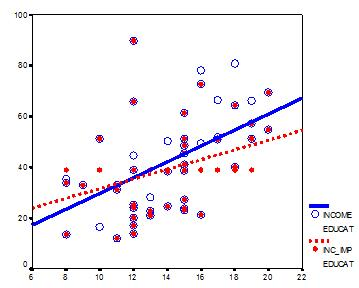# The First Problem with Mean Imputation

by

I’m on a mission to warn the innocent of the dangers of mean imputation.

Perhaps that’s a bit dramatic, but mean imputation (also called mean substitution) really ought to be a last resort in most situations.  There are many alternatives to mean imputation that provide much more accurate parameter estimates and standard errors, so there really is no excuse to use it.

This post is the first in a series explaining the many reasons not to use mean imputation (and to be fair, its advantages).

First, a definition: mean imputation is the replacement of a missing observation with the mean of the non-missing observations for that variable.

### Problem #1: Mean imputation does not preserve the relationships among variables.

True, imputing the mean preserves the mean of the observed data.  So if the data are missing completely at random, the estimate of the mean remains unbiased. Plus, by imputing the mean, you are able to keep your sample size up to the full sample size.

This is the original logic involved in mean imputation.

If all you are doing is estimating means (which is rarely the point of research studies), mean imputation will not bias your parameter estimate (if the data are missing completely at random).

It will still bias your standard error, but I will get to that in another post.

Since most research studies are interested in the relationship among variables, mean imputation is not a good solution.  The following graph illustrates this well:This graph illustrates hypothetical data between X=years of education and Y=annual income in thousands with n=50.  The blue circles are the original data, and the solid blue line indicates the best fit regression line for the full data set.  The correlation between X and Y is r=.53.

I then randomly deleted 12 observations of income (Y) and substituted the mean.  The dataset with the mean imputations is shown by the red dots.

Blue circles with red dots inside them represent non-missing data.  Blue circles that are empty represent the missing data.   If you look across the graph at Y=39, you will see a row of red dots without blue circles.  These represent the imputed values.

The dotted red line represents the new best fit regression line with the imputed data.  As you can see, it is less steep than the original line, and the new correlation is now r=.39.  It is still significant, but it is clearly biased toward 0.

The real relationship is quite underestimated.

One note: if X were missing instead of Y, mean substitution would artificially inflate the correlation.

In other words, you’ll think there is a stronger relationship than there really is.Approaches to Missing Data: the Good, the Bad, and the Unthinkable
Learn the different methods for dealing with missing data and how they work in different missing data situations.

### Related PostsQueen sheba

am carrying out a research on mean imputation and random imputation.to know which one is more efficientAndreea

any examples of papers that have used mean imputation out of curiosity? It is said many researchers still used this method so I’d be curious to see such examples. ThanksMar

Hi
This may sound very basic but how do we conduct a mean imputation in spss?

MarKaren

Hi Mar,

Many procedures have a checkbox, but I have to say, most of the time mean imputation causes more problems than it solves. There are many, many better approaches.Revathi sathish

Hi,
It s good explanation. can you tell me about hot deck, cold deck, deductive, cell mean imputations advantage and disadvantage.Karen